# Conjunction

## Learn About Conjunction With The Following Examples And Interactive Exercises.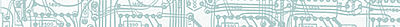Example 1:

 Given: p: Ann is on the softball team. q: Paul is on the football team. Problem: What does p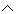q represent?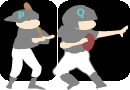Solution: In Example 1, statement p represents the sentence, "Ann is on the softball team," and statement q represents the sentence, "Paul is on the football team." The symbolis a logical connector which means "and." Therefore, the compound statement pq represents the sentence, "Ann is on the softball team and Paul is on the footballteam." The statement pq is a conjunction.

Definition: conjunction is a compound statement formed by joining two statements with the connector AND. The conjunction "p and q" is symbolized by pq. A conjunction is true when both of its combined parts are true; otherwise it is false.

Now that we have defined a conjunction, we can apply it to Example 1. The conjunction pq is true when both "Ann is on the softball team" and "Paul is on the football team" are true statements; otherwise it is false. We can construct a truth table for the conjunction "p and q." In order to list all truth values of pq, we start by listing every combination of truth values in the first two columns of the truth table below.

 p q pq T T T F F T F F

Next, we complete the last column according to the rules for conjunction listed above.

 p q pq T T T T F F F T F F F F

The truth table above lists the truth values of pq. A truth table is an excellent tool for listing the truth values of a conjunction (or any compound statement). (Note: Throughout our lessons on symbolic logic, we will always construct truth tables with the first two columns listed exactly as above. The order of the truth values in these first columns is critical to finding all truth values for a given statement. This order will also apply to other formats used to list truth values in more advanced lessons.) Let's look at some more examples of conjunction.

Example 2:

 Given: a: A square is a quadrilateral. b: Harrison Ford is an American actor. Problem: Construct a truth table for the conjunction "a and b."Solution:

 a b ab T T T T F F F T F F F F

Example 3:

 Given: r: The number x is odd. s: The number x is prime. Problem: Can we list all truth values for rs in a truth table?Why or why not?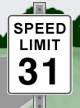Solution: Since each statement given in this example represents an open sentence, the truth value of rs will depend on the value of variable x. But there are an infinite number of replacement values for x, so we cannot list all truth values for rs in a truth table. We can, however, find the truth value of rs for given values of x as shown below.

If x = 3, then r is true, s is true. The conjunction rs is true.

If x = 9, then r is true, s is false. The conjunction rs is false.

If x = 2, then r is false, s is true. The conjunction rs is false.

If x = 6, then r is false, s is false. The conjunction rs is false.

In the next example we are given the truth values of each statement. We are then asked to determine the truth values of the specified conjunctions.

Example 4:

 Given: p: The number 11 is prime. true q: The number 17 is composite. false r: The number 23 is prime. true Problem: For each conjunction below, write a sentence and indicate if it is true or false.
 1 pq The number 11 is prime and the number 17 is composite. false 2 pr The number 11 is prime and the number 23 is prime. true 3 qr The number 17 is composite and the number 23 is prime. false

A conjunction is formed by combining two statements with the connector "and." One of these statements can be a negation as shown in the example below.

Example 5: Construct a truth table for each conjunction below:

 1 x and y 2 ~x and y 3 ~y and x

Solution:

 x y xy T T T T F F F T F F F Fx y ~x ~xy T T F F T F F F F T T T F F T Fx y ~y ~yx T T F F T F T T F T F F F F T F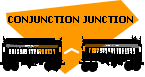Summary: A conjunction is a compound statement formed by joining two statements with the connector "and." The conjunction "p and q" is symbolized by pq. A conjunction is true when both of its combined parts are true; otherwise it is false.

### Exercises

Directions: Read each question below. Select your answer by clicking on its button. Feedback to your answer is provided in the RESULTS BOX. If you make a mistake, choose a different button.

 1. Which of the following sentences is a conjunction? Jill eats pizza or Sam eats pretzels.Jill eats pizza but not pretzels.Jill eats pizza and Sam eats pretzels.None of the above. RESULTS BOX:
 2. Which of the following statements is a conjunction? p + qpq~pNone of the above. RESULTS BOX:
 3. A conjunction is used with which connector? NotOrAndNone of the above. RESULTS BOX:
 4. If a is false and b is true, what is the truth value of ab? TrueFalseNot enough information was given.None of the above. RESULTS BOX:
5.
 Given: r: y is prime. s: y is even. Problem: What is the truth value of rs when y is replaced by 2?

True
False
Not enough information was given.
None of the above.

RESULTS BOX:

 Lessons on Symbolic Logic Negation Conjunction Disjunction Conditional Compound Biconditional Tautologies Equivalence Practice Exercises Challenge Exercises Solutions